Documentation

### This is machine translation

Mouseover text to see original. Click the button below to return to the English version of the page.

Note: This page has been translated by MathWorks. Click here to see
To view all translated materials including this page, select Country from the country navigator on the bottom of this page.

# sim

Simulate response of identified model

## Syntax

``y = sim(sys,udata)``
``y = sim(sys,udata,opt)``
``[y,y_sd] = sim(___)``
``````[y,y_sd,x] = sim(___)``````
``[y,y_sd,x,x_sd] = sim(___)``
``sim(___)``

## Description

example

````y = sim(sys,udata)` returns the simulated response of an identified model using the input data, `udata`. By default, zero initial conditions are used for all model types except `idnlgrey`, in which case the initial conditions stored internally in the model are used.```

example

````y = sim(sys,udata,opt)` uses the option set, `opt`, to configure the simulation option, including the specification of initial conditions.```

example

````[y,y_sd] = sim(___)` returns the estimated standard deviation, `y_sd`, of the simulated response. ```

example

``````[y,y_sd,x] = sim(___)``` returns the state trajectory, `x`, for state-space models.```

example

````[y,y_sd,x,x_sd] = sim(___)` returns the standard deviation of the state trajectory, `x_sd`, for state-space models.```

example

````sim(___)` plots the simulated response of the identified model.```

## Examples

collapse all

Load the estimation data.

`load iddata2 z2`

Estimate a third-order state-space model.

`sys = ssest(z2,3);`

Simulate the identified model using the input channels from the estimation data.

`y = sim(sys,z2);`

Load the data, and obtain the identified model.

```load iddata2 z2 sys = n4sid(z2,3);```

`sys` is a third-order state-space model estimated using a subspace method.

Create a simulation option set to add noise to the simulated model response.

`opt1 = simOptions('AddNoise',true);`

Simulate the model.

`y = sim(sys,z2,opt1);`

Default Gaussian white noise is filtered by the noise transfer function of the model and added to the simulated model response.

You can also add your own noise signal, `e`, using the `NoiseData` option.

```e = randn(length(z2.u),1); opt2 = simOptions('AddNoise',true,'NoiseData',e);```

Simulate the model.

`y = sim(sys,z2,opt2);`

Load data.

`load iddata1 z1`

Specify the estimation option to estimate the initial state.

`estimOpt = ssestOptions('InitialState','estimate');`

Estimate a state-space model, and return the value of the estimated initial state.

`[sys,x0] = ssest(z1,2,estimOpt);`

Specify initial conditions for simulation

`simOpt = simOptions('InitialCondition',x0);`

Simulate the model, and obtain the model response and standard deviation.

`[y,y_sd] = sim(sys,z1,simOpt);`

Load estimation data, and estimate a state-space model.

```load iddata1 z1 sys = ssest(z1,2);```

Return the standard deviation and state trajectory.

`[y,y_sd,x] = sim(sys,z1);`

Load estimation data, and estimate a state-space model.

```load iddata1 z1 sys = ssest(z1,2);```

Create a simulation option set, and specify the initial states.

`opt = simOptions('InitialCondition',[1;2]);`

Specify the covariance of the initial states.

`opt.X0Covariance = [0.1 0; 0 0.1];`

Calculate the standard deviations of simulated response, `y_sd`, and state trajectory, `x_sd`.

`[y,y_sd,x,x_sd] = sim(sys,z1,opt);`

Obtain the identified model.

```load iddata2 z2 sys = tfest(z2,3);```

`sys` is an `idtf` model that encapsulates the third-order transfer function estimated for the measured data `z2`.

Simulate the model.

`sim(sys,z2)`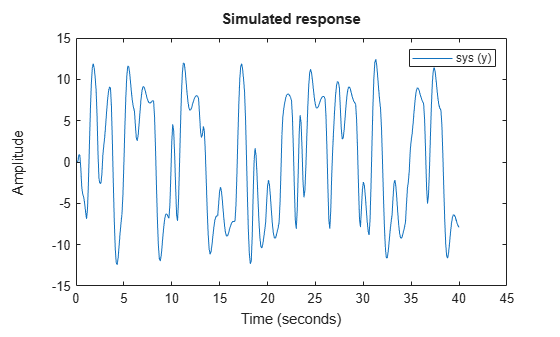Simulate a single-input single-output nonlinear ARX model around a known equilibrium point, with an input level of `1` and output level of `10`.

Load the sample data.

`load iddata2`

Estimate a nonlinear ARX model from the data.

`M = nlarx(z2,[2 2 1],'treepartition');`

Estimate current states of model based on past data. Specify as many past samples as there are lags in the input and output variables (2 here).

`x0 = data2state(M,struct('Input',ones(2,1),'Output',10*ones(2,1)));`

Simulate the model using the initial states returned by `data2state`.

```opt = simOptions('InitialCondition',x0); sim(M,z2,opt)```Continue the simulation of a nonlinear ARX model from the end of a previous simulation run.

Estimate a nonlinear ARX model from data.

```load iddata2 M = nlarx(z2,[2 2 1],'treepartition');```

Simulate the model using the first half of the input data `z2`. Start the simulation from zero initial states.

```u1 = z2(1:200,[]); opt1 = simOptions('InitialCondition','zero'); ys1 = sim(M,u1,opt1);```

Start another simulation using the second half of the input data `z2`. Use the same states of the model from the end of the first simulation.

`u2 = z2(201:end,[]);`

To set the initial states for the second simulation correctly, package input `u1` and output `ys1` from the first simulation into one `iddata` object. Pass this data as initial conditions for the next simulation.

```firstSimData = [ys1,u1]; opt2 = simOptions('InitialCondition',firstSimData); ys2 = sim(M,u2,opt2);```

Verify the two simulations by comparing to a complete simulation using all the input data `z2`. First, extract the whole set of input data.

```uTotal = z2(:,[]); opt3 = simOptions('InitialCondition','zero'); ysTotal = sim(M,uTotal,opt3);```

Plot the three responses `ys1`, `ys2` and `ysTotal.` `ys1` should be equal to first half of `ysTotal`. `ys2` should be equal to the second half of `ysTotal`.

`plot(ys1,'b',ys2,'g',ysTotal,'k*')`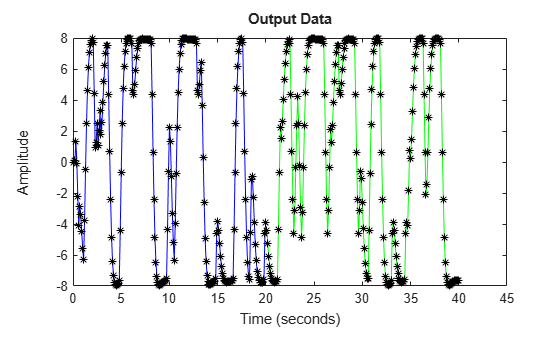The plot shows that the three responses `ys1`, `ys2`, and `ysTotal` overlap as expected.

Estimate initial states of model `M` such that, the response best matches the output in data set `z2`.

Load the sample data.

`load iddata2;`

Estimate a nonlinear ARX model from the data.

`M = nlarx(z2,[4 3 2],wavenet('NumberOfUnits',20));`

Estimate the initial states of `M` to best fit `z2.y` in the simulated response.

`x0 = findstates(M,z2,Inf);`

Simulate the model.

```opt = simOptions('InitialCondition',x0); ysim = sim(M,z2.u,opt);```

Compare the simulated model output `ysim` with the output signal in `z2`.

```time = z2.SamplingInstants; plot(time,ysim,time,z2.y,'.')```Start simulation of a model near steady state, where the input is known to be `1`, but the output is unknown.

Load the sample data.

`load iddata2`

Estimate a nonlinear ARX model from the data.

`M = nlarx(z2,[4 3 2],'wavenet');`

Determine equilibrium state values for input `1` and unknown target output.

`x0 = findop(M,'steady',1, NaN);`

Simulate the model using initial states `x0`.

```opt = simOptions('InitialCondition',x0); sim(M,z2.u,opt)```Load the sample data.

`load iddata2`

Create a Hammerstein-Wiener model.

`M = nlhw(z2,[4 3 2],[],'pwlinear');`

Compute steady-state operating point values corresponding to an input level of `1` and an unknown output level.

`x0 = findop(M,'steady',1,NaN);`

Simulate the model using the estimated initial states.

```opt = simOptions('InitialCondition',x0); sim(M,z2.u)```Load time series data, and estimate an AR model using the least-squares approach.

```load iddata9 z9 sys = ar(z9,6,'ls');```

For time series data, specify the desired simulation length, N = 200 using an N-by-0 input data set.

`data = iddata([],zeros(200,0),z9.Ts);`

Set the initial conditions to use the initial samples of the time series as historical output samples.

```IC = struct('Input',[],'Output',z9.y(1:6)); opt = simOptions('InitialCondition',IC);```

Simulate the model.

`sim(sys,data,opt)`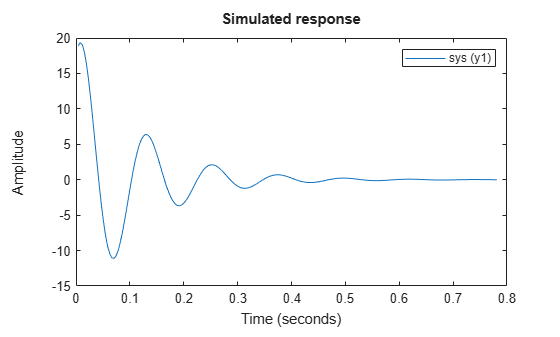Use historical input-output data as a proxy for initial conditions when simulating your model. You first simulate using the `sim` command and specify the historical data using the `simOptions` option set. You then reproduce the simulated output by manually mapping the historical data to initial states.

Load a two-input, one-output data set.

`load iddata7 z7`

Identify a fifth-order state-space model using the data.

`sys = n4sid(z7,5);`

Split the data set into two parts.

```zA = z7(1:15); zB = z7(16:end);```

Simulate the model using the input signal in `zB`.

`uSim = zB;`

Simulation requires initial conditions. The signal values in `zA` are the historical data, that is, they are the input and output values for the time immediately preceding data in `zB`. Use `zA` as a proxy for the required initial conditions.

```IO = struct('Input',zA.InputData,'Output',zA.OutputData); opt = simOptions('InitialCondition',IO);```

Simulate the model.

`ysim = sim(sys,uSim,opt);`

Now reproduce the output by manually mapping the historical data to initial states of `sys`. To do so, use the `data2state` command.

`xf = data2state(sys,zA);`

`xf` contains the state values of `sys` at the time instant immediately after the most recent data sample in `zA`.

Simulate the system using `xf` as the initial states.

```opt2 = simOptions('InitialCondition',xf); ysim2 = sim(sys,uSim,opt2);```

Plot the output of the `sim` command `ysim` and the manually computed results `ysim`2.

`plot(ysim,'b',ysim2,'--r')`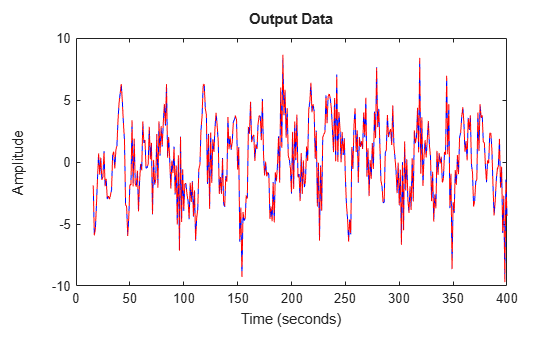`ysim2` is the same as `ysim`.

## Input Arguments

collapse all

Identified model, specified as one of the following model objects:

Model TypeModel Object
Identified Linear ModelPolynomial model`idpoly`
Process model`idproc`
State-space model`idss`
Transfer function model`idtf`
Linear grey-box model`idgrey`
Identified Nonlinear ModelNonlinear ARX model`idnlarx`
Nonlinear Hammerstein-Wiener model`idnlhw`
Nonlinear grey-box model`idnlgrey`

Simulation input data, specified as an `iddata` object or a matrix. `sim` uses the input channels from this object as the simulation inputs. For time-domain simulation of discrete-time systems, you can also specify `udata` as a matrix with columns that correspond to each input channel.

If `sys` is a linear model, you can use either time-domain or frequency-domain data. If `sys` is a nonlinear model, you can only use time-domain data.

If `sys` is a time-series model, that is a model with no inputs, specify `udata` as an Ns-by-0 signal, where Ns is the wanted number of simulation output samples. For example, to simulate `100` output samples, specify `udata` as follows.

`udata = iddata([],zeros(100,0),Ts);`

If you do not have data from an experiment, use `idinput` to generate signals with various characteristics.

Simulation options, specified as a `simOptions` option set for setting the following options:

• Initial conditions

• Input/output offsets

• Additive noise

## Output Arguments

collapse all

Simulated response for `sys`, returned as an `iddata` object or matrix, depending on how you specify `udata`. For example, if `udata` is an `iddata` object, then so is `y`.

If `udata` represents time-domain data, then `y` is the simulated response for the time vector corresponding to `udata`.

If `udata` represents frequency-domain data, U(ω), then `y` contains the Fourier transform of the corresponding sampled time-domain output signal. This signal is the product of the frequency response of `sys`, G(ω), and U(ω).

For multi-experiment data, `y` is a corresponding multi-experiment `iddata` object.

Estimated standard deviation of the simulated response for linear models or nonlinear grey-box models, returned as an Ns-by-Ny matrix, where Ns is the number of samples and Ny is the number of outputs. The software computes the standard deviation by taking into account the model parameter covariance, initial state covariance, and additive noise covariance. The additive noise covariance is stored in the `NoiseVariance` property of the model.

`y_sd` is derived using first order sensitivity considerations (Gauss approximation formula).

For nonlinear models, `y_sd` is `[]`.

Estimated state trajectory for state-space models, returned as an Ns-by-Nx matrix, where Ns is the number of samples and Nx is the number of states.

`x` is only relevant if `sys` is an `idss`, `idgrey`, or `idnlgrey` model. If `sys` is not a state-space model, `x` is returned as `[]`.

Estimated standard deviation of state trajectory for state-space models, returned as an Ns-by-Nx matrix, where Ns is the number of samples and Nx is the number of states. The software computes the standard deviation by taking into account the model parameter covariance, initial state covariance, and additive noise covariance. The additive noise covariance is stored in the `NoiseVariance` property of the model.

`x_sd` is only relevant if `sys` is an `idss`, `idgrey`, or `idnlgrey` model. If `sys` is not a state-space model, `x_sd` is returned as `[]`.

## Tips

• When the initial conditions of the estimated model and the system that measured the validation data set are different, the simulated and measured responses may also differ, especially at the beginning of the response. To minimize this difference, estimate the initial state values using `findstates` and use the estimated values to set the `InitialCondition` option using `simOptions`. For an example, see Match Model Response to Output Data.

## Algorithms

Simulation means computing the model response using input data and initial conditions. `sim` simulates the following system: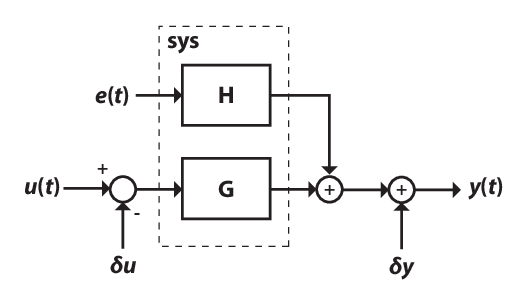Here,

• u(t) is the simulation input data, `udata`.

• y(t) is the simulated output response.

• G is the transfer function from the input to the output and is defined in `sys`. The simulation initial conditions, as specified using `simOptions`, set the initial state of G.

• e(t) is an optional noise signal. Add noise to your simulation by creating a `simOptions` option set, and setting the `AddNoise` option to `true`. Additionally, you can change the default noise signal by specifying the `NoiseData` option.

• H is the noise transfer function and is defined in `sys`.

• δu is an optional input offset subtracted from the input signal, u(t), before the input is used to simulate the model. Specify an input offset by setting the `InputOffset` option using `simOptions`.

• δy is an optional output offset added to the output response, y(t), after simulation. Specify an output offset by setting the `OutputOffset` option using `simOptions`.

For more information on specifying simulation initial conditions, input and output offsets, and noise signal data, see `simOptions`. For multiexperiment data, you can specify these options separately for each experiment.

## Alternatives

• Use `simsd` for a Monte-Carlo method of computing the standard deviation of the response.

• `sim` extends `lsim` to facilitate additional features relevant to identified models:

• Simulation of nonlinear models

• Simulation with additive noise

• Incorporation of signal offsets

• Computation of response standard deviation (linear models only)

• Frequency-domain simulation (linear models only)

• Simulations using different intersample behavior for different inputs

To obtain the simulated response without any of the preceding operations, use `lsim`.

## See Also

### Topics

#### Introduced before R2006a

##### SupportGet trial now